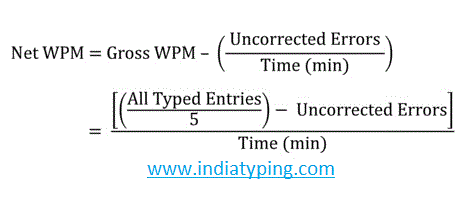# Formula for Calculating Typing Speed

For calculating Typing Speed there are two formulas are used. One formula is based upon characters counting and the second one is directly applied on words count. Let explore the two typing test speed calculation formulas in detail for Hindi Typing and English Typing test.

## 1. Character based Formula

It's the international standard formula for typing test. This formula is independent from exercise text. Means give a justified result with any passage your are typing.

A . Gross WPMHere WPM = Word Per Minute

All typed entries = Total Key Strokes (or key depression)

Gross WPM is really the typing speed that you typed in one minute its getting by total key Stroke (Key depression) divided by 5 (One word = 5 character) and then divided by total minute to get word per minute speed.

If you type 200 character/keystroke in 1 minute then your typing speed will be = 200 / 5 = 40 WPM

But GWPM is not your actual speed, actual speed is calculating by correct word you typed known as 'Net WPM'.

Note: delete, backspace, shift and other modifier keys are not calculated in key stroke. Space between two word's also counted as 1 character or keystroke.

B. Net WPM FormulaCalculation of Net WPM

Net speed can be calculated by deducting errors in gross wpm. For example if you typed 40 Word in 1 Minute but you typed 3 word wrong then your Net WPM = 40 - 3 = 37 WPM

C. Accuracy Formula:

Accuracy is a percentage ratio of Gross and Net Word Speed:

Accuracy = (Net WPM / Gross WPM ) * 100 = 37 / 40 * 100 = 92.5 %

## 2. Words based formula :

In word base calculation a set of characters seprated by space considar as a word. The word count regardless of how many characters in it. For Example the sentence "We are learning typing."  As you can see here total 4 words. These formula applied only when the all candidate have take test for same exercise. If the exercise is different for different batch's taking test then the result is not consider justified with this formula.

A. Gross WPM : Total Words Typed / Total Time Taken (in minutes)

Example :  Suppose a candidate A Typed total 350 Words in 10 Minutes:

Gross Speed in WPM : 350/10 = 35 WPM

B. Net Speed : (Total Words Typed - Word Error ) / Total Time Taken (in minutes)

Example : Suppose the A candidate typed total 350 words in 10 Minutes in which he typed 20 words wrong:

Net Speed in WPM = (350 -20) / 10 = 33 WPM

C. Accuracy : (Net WPM/ Gross WPM ) * 100

Accuracy = 33/35 * 100 = 94.28 %

### Calculation of Errors

Errors are calculated by following two criteria
1. Errors which are corrected

If you correct wrong word that you typed then you will use backspace to delete that word will not calculated in WPM.

2. Errors which are incorrect

If you not correct the errors you made then you will penalized 5 character for each word error (Regardless of how many character in this wrong word). So always try to type accurate and if mistake made then correct it.

## What is a Qualifying test ?

Qualifying typing test required a minimum speed criteria with permissible errors. For example 40 WPM speed with 5% error perdmissible. In this example if you achieve 40 WPM speed and make errors <= 5% than you will consider qualified in exam. Here 40 WPM is the GWPM as described above.

### What is the typing score calculation formula for SSC typing test ?

SSC uses their own customize formula, the formula is same as above but the calculation of errors is different for SSC exams. You can check evaluation of typing test in SSC. For SSC typing test practice

### What is the typing score calculation formula for CPCT typing test ?

CPCT uses the character based formula given above you can check evaluation of CPCT typing Score in details.

Use our English to Hindi Translation, to convert text written in English to Hindi with accuracy